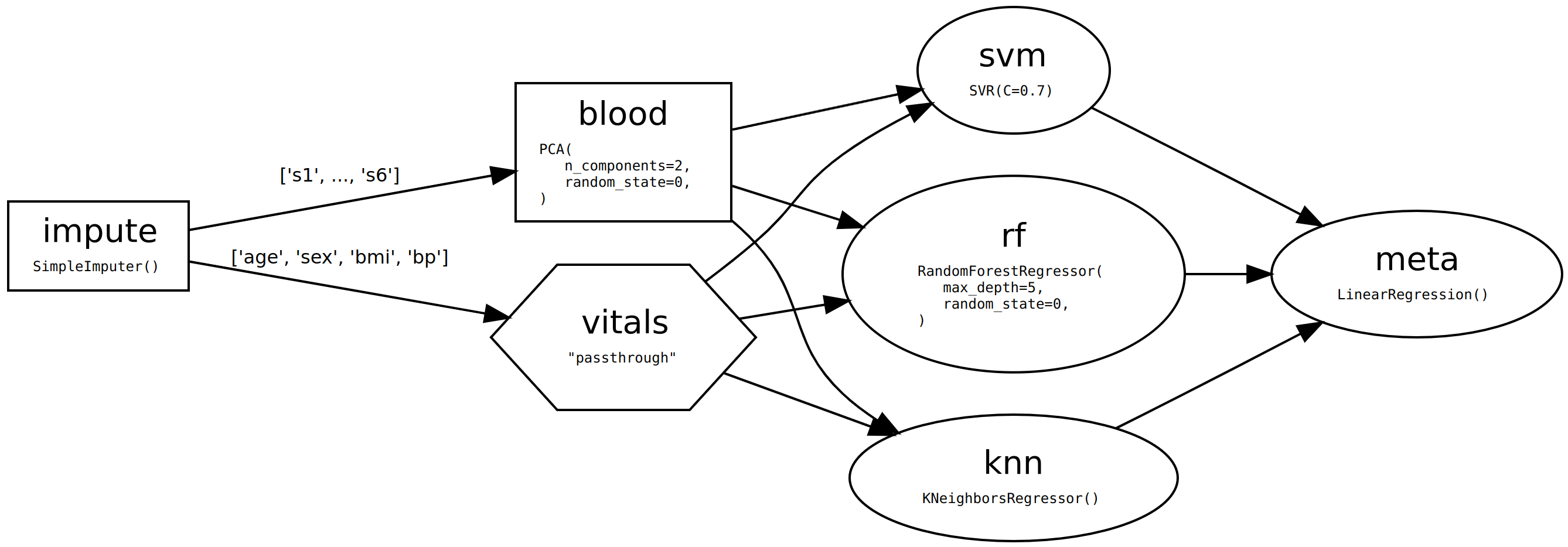# skdag - scikit-learn workflow management¶

scikit-dag (`skdag`) is an open-sourced, MIT-licenced library that provides advanced workflow management to any machine learning operations that follow `sklearn` conventions. It does this by introducing Directed Acyclic Graphs (`skdag.dag.DAG`) as a drop-in replacement for traditional scikit-learn `sklearn.pipeline.Pipeline`. This gives you a simple interface for a range of use cases including complex pre-processing, model stacking and benchmarking.

```from skdag import DAGBuilder

dag = (
DAGBuilder(infer_dataframe=True)
"vitals",
"passthrough",
deps={"impute": ["age", "sex", "bmi", "bp"]},
)
"blood",
PCA(n_components=2, random_state=0),
deps={"impute": ["s1", "s2", "s3", "s4", "s5", "s6"]},
)
"rf",
RandomForestRegressor(max_depth=5, random_state=0),
deps=["blood", "vitals"],
)
.add_step("svm", SVR(C=0.7), deps=["blood", "vitals"])
"knn",
KNeighborsRegressor(n_neighbors=5),
deps=["blood", "vitals"],
)
.add_step("meta", LinearRegression(), deps=["rf", "svm", "knn"])
.make_dag(n_jobs=2, verbose=True)
)

dag.show(detailed=True)
```The above DAG imputes missing values, runs PCA on the columns relating to blood test results and leaves the other columns as they are. Then they get passed to three different regressors before being passed onto a final meta-estimator. Because DAGs (unlike pipelines) allow predictors in the middle or a workflow, you can use them to implement model stacking. We also chose to run the DAG steps in parallel wherever possible.

After building our DAG, we can treat it as any other estimator:

```from sklearn import datasets

X, y = datasets.load_diabetes(return_X_y=True, as_frame=True)
X_train, X_test, y_train, y_test = train_test_split(
X, y, test_size=0.2, random_state=0
)

dag.fit(X_train, y_train)
dag.predict(X_test)
```

Just like a pipeline, you can optimise it with a gridsearch, pickle it etc.

Note that this package does not deal with things like delayed dependencies and distributed architectures - consider an established solution for such use cases. `skdag` is just for building and executing local ensembles from estimators.

Read on to learn more about `skdag`

## Getting started¶

A practical introduction to DAGs for scikit-learn.

## User Guide¶

Details of the full functionality provided by `skdag`.

## API Documentation¶

Detailed API documentation.

## Examples¶

Further examples that complement the User Guide.